## 摘要

Multi-gate Mixture-of-Experts (MMoE)通过在多任务学习中引入Mixture-of-Experts（MoE）层，显式的学习了各个子任务之间关系，同时利用门限网络以优化每个任务。

## Motivation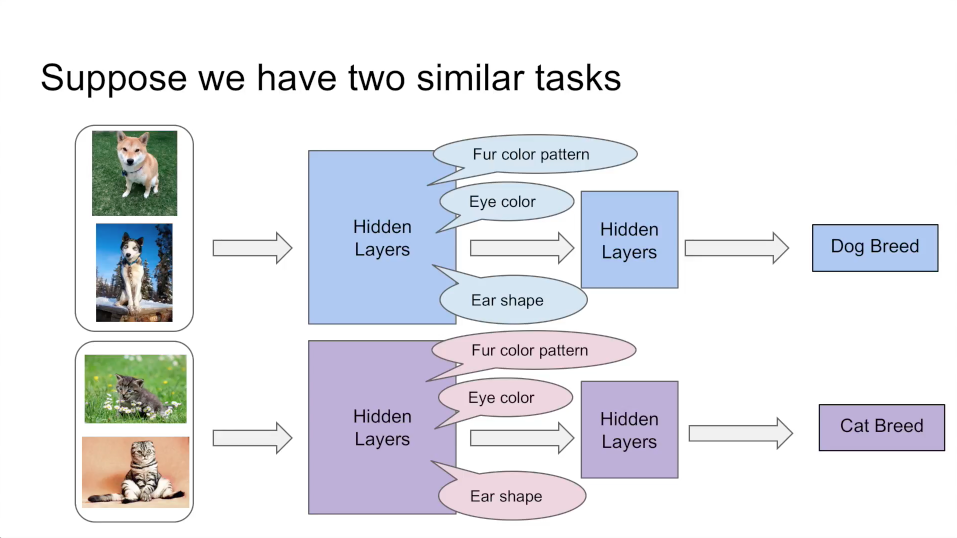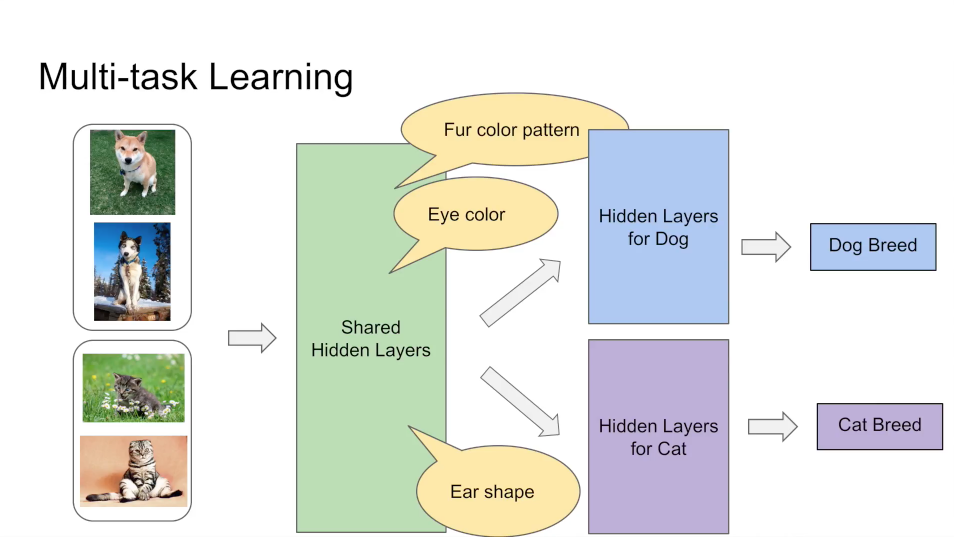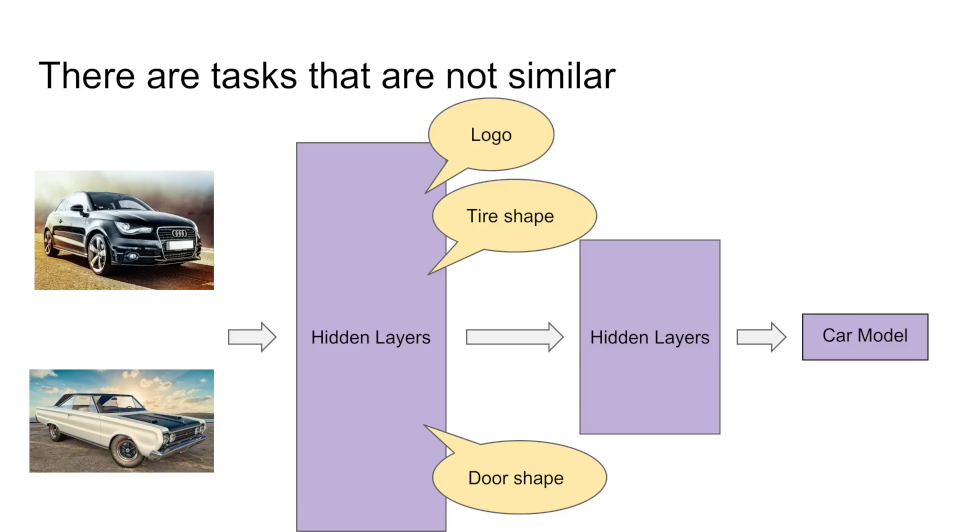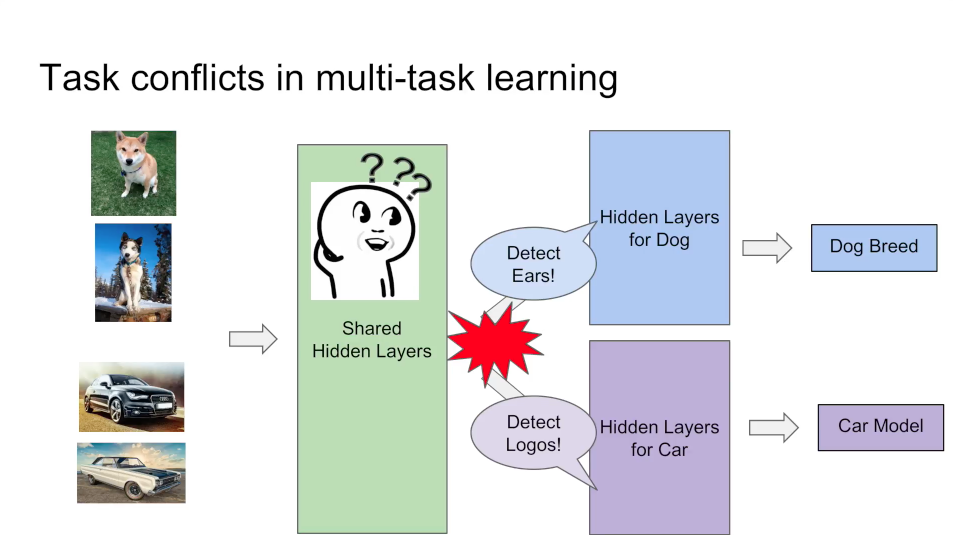• 有一些方案通过假设每个任务的特定数据生成过程，根据假设度量各个任务的差异，然后根据任务差异为后续多任务训练提出指导意见，但是，由于实际应用中数据模式是很复杂的，度量任务之间的差异并且把对应的指导意见利用起来是很困难的。
• 有一些方案可以不依赖任务差异度量的情况下处理多任务学习过程中的差异，但是这些方法通常会为每个模型增加更多模型参数，导致计算开销变大。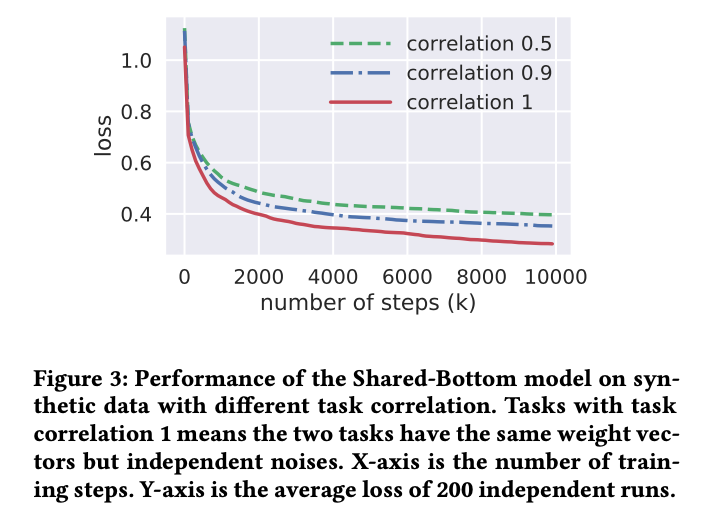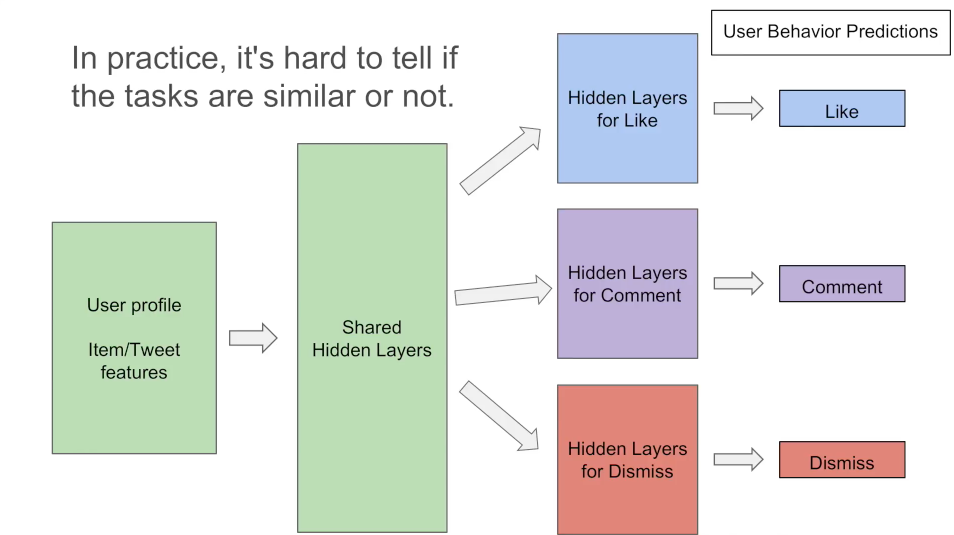## Multi-gate Mixture-of-Experts(MMoE)

We want the model itself to figure out to which extent to share among tasks.

### Mixture-of-Experts(MoE)

MoE Model

$y=\sum_{i=1}^{n} g(x)_{i} f_{i}(x)$

• $\sum_{i=1}^{n} g(x)_{i}=1$，其中$g(x)_{i}$表示专家$f_i$的权重，是做过归一化的。g代表一个汇总所有专家结果的门控网络。
• $f_{i}, i=1, \ldots, n$，表示n个expert networks
• 门控网络根据输入生成n位专家的分布，最终输出为所有专家输出的加权总和。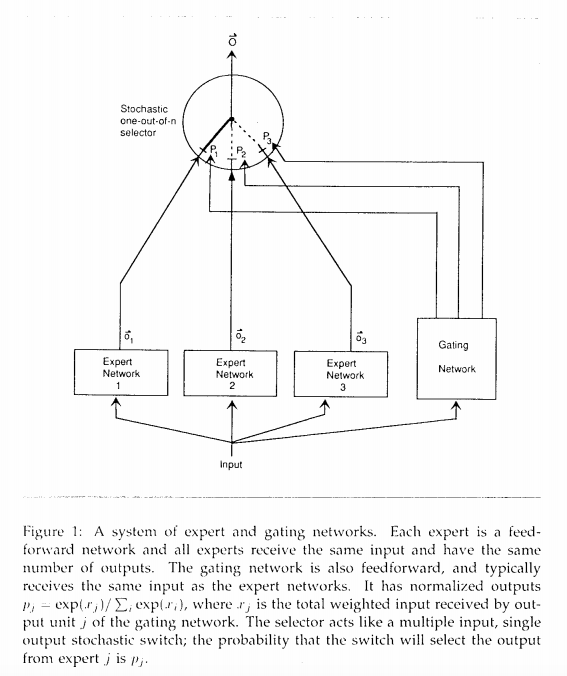MoE Layer

• MoE Layer具有与MoE Model相同的结构，但接受前一层的输出作为输入，并输出到连续的层。然后以端到端的方式训练整个模型。
• MoE Layer结构的主要目标是实现条件计算，其中每个实例仅激活部分网络。 对于每个输入示例，模型都可以通过以输入为条件的门控网络来选择专家的子集。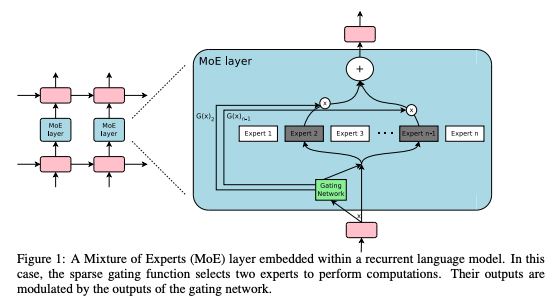### MMoE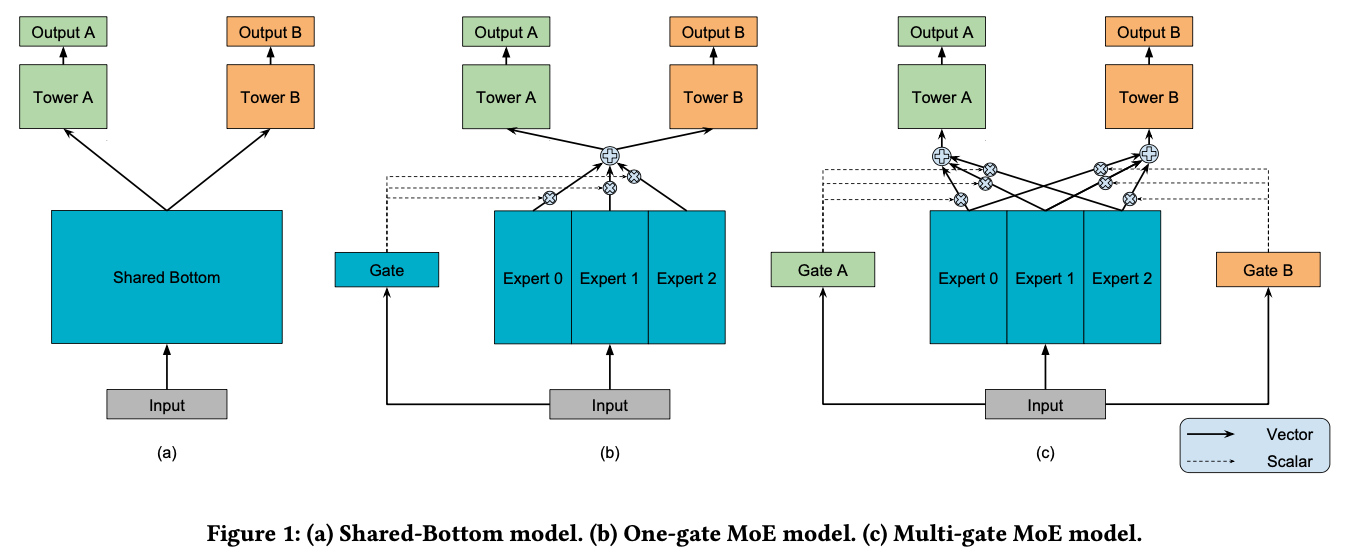$y_{k}=h^{k}(f(x))$

• $k$表示k个任务
• $f$表示Shared-Bottom网络
• $h^k$表示每个子任务对应的Tower Network

OMoE是在多任务学习中引入MoE层，将input分别输入给三个Expert(共享子网络)，但Expert并不共享参数。同时将input输出给Gate(门限网络)，Gate输出每个Expert被选择的概率，然后将三个Expert的输出加权求和，输出给Tower，有点attention的感觉。函数表达式为：

$y^{k}=h^{k}\left(\sum_{i=1}^{n} g_{i} f_{i}(x)\right)$

• $n$表示n个专家网络

OMoE的主要目标是实现条件计算，对于每个数据而言，只有部分网络是活跃的，该模型可以通过限制输入的门控网络来选择专家网络的子集。

MMoE的目的在于捕获任务差异，而与共享底部多任务模型相比，不需要明显增加更多的模型参数。底部引入MoE层，来显式的对多个任务的关系进行建模，或者理解成学习所有任务的不同方面；再对每个任务学习一个Gate(门限网络)，这个Gate可以理解成这个任务在各个方面的特点。函数表达式为：

\begin{aligned} y_{k} &=h^{k}\left(f^{k}(x)\right) \\ \text { where } f^{k}(x) &=\sum_{i=1}^{n} g^{k}(x)_{i} f_{i}(x) . \end{aligned}

MMoE的每个Gate网络都可以根据不同任务来选择专家网络的子集，所以即使两个任务并不是十分相关，那么经过 Gate 后也可以得到不同的权重系数，此时，MMoE 可以充分利用部分 expert 网络的信息，近似于单个任务；而如果两个任务相关性高，那么Gate的权重分布相差会不大，会类似于一般的多任务学习。

## 实验结果

Performance on Data with Different Task Correlations

• 对于所有模型，具有较高相关性的数据的效果要优于具有较低相关性的数据的效果；
• 在两个任务相关性搞的情况下，MMoE模型和OMoE模型之间的效果几乎没有区别；但是，当任务之间的相关性降低时，OMoE模型的效果就会明显下降，而对MMoE模型的影响却很小。因此，在低关联性情况下，具有特定于任务的门来建模任务差异至关重要；
• OMoE和MMoE的效果在不同相关度任务的数据中都好于Shared-Bottom。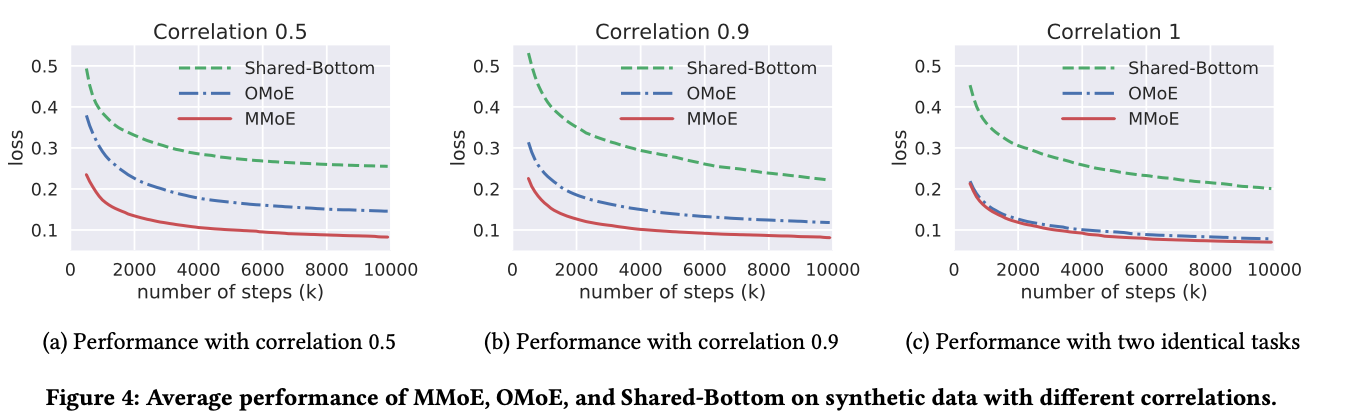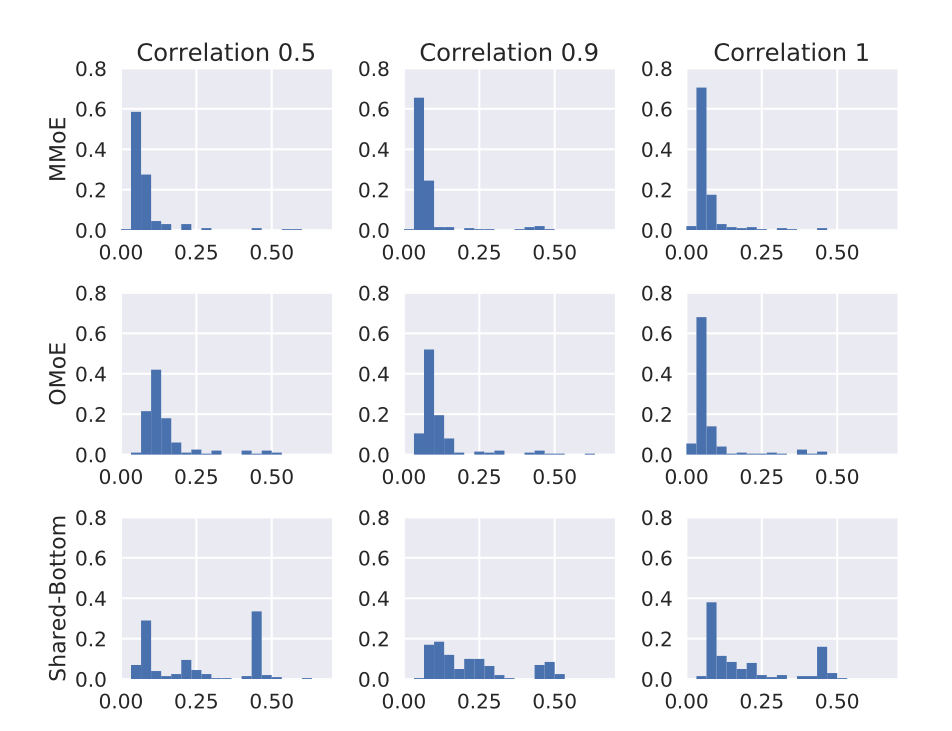• 首先，在所有任务相关性设置中，Shared-Bottom模型的性能差异远大于基于MoE的模型的性能差异。这意味着，与基于MoE的模型相比，Shared-Bottom模型通常具有质量较差的局部最小值。
• 其次，虽然任务相关性为1时OMoE模型的性能方差与MMoE模型相似，但当任务相关性降低到0.5时，OMoE的鲁棒性却明显下降。这验证了multi-gate结构在解决由任务差异引起的冲突而导致的不良局部最小值方面的有用性。
• 最后，这三个模型中的最低loss是可比的。具有足够的模型容量，应该存在一个“正确”的 Shared-Bottom模型，该模型可以很好地学习这两个任务。